Warning

This documents an unmaintained version of NetworkX. Please upgrade to a maintained version and see the current NetworkX documentation.

# geographical_threshold_graph¶

geographical_threshold_graph(n, theta, alpha=2, dim=2, pos=None, weight=None)[source]

Returns a geographical threshold graph.

The geographical threshold graph model places n nodes uniformly at random in a rectangular domain. Each node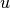is assigned a weight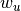. Two nodesand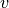are joined by an edge if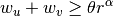where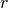is the Euclidean distance betweenand, and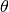,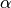are parameters.

Parameters: n (int) – Number of nodes theta (float) – Threshold value alpha (float, optional) – Exponent of distance function dim (int, optional) – Dimension of graph pos (dict) – Node positions as a dictionary of tuples keyed by node. weight (dict) – Node weights as a dictionary of numbers keyed by node. Graph

Examples

>>> G = nx.geographical_threshold_graph(20, 50)


Notes

If weights are not specified they are assigned to nodes by drawing randomly from the exponential distribution with rate parameter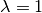. To specify weights from a different distribution, use the weight keyword argument:

>>> import random
>>> n = 20
>>> w = {i: random.expovariate(5.0) for i in range(n)}
>>> G = nx.geographical_threshold_graph(20, 50, weight=w)


If node positions are not specified they are randomly assigned from the uniform distribution.

References

  Masuda, N., Miwa, H., Konno, N.: Geographical threshold graphs with small-world and scale-free properties. Physical Review E 71, 036108 (2005)
  Milan Bradonjić, Aric Hagberg and Allon G. Percus, Giant component and connectivity in geographical threshold graphs, in Algorithms and Models for the Web-Graph (WAW 2007), Antony Bonato and Fan Chung (Eds), pp. 209–216, 2007